cancel
Showing results for
Did you mean:Helper III

## Slicer DAX

1) I am trying to get all my options in the slicer to show values. Currently, I can only see values in the cluster column visual for “Y”: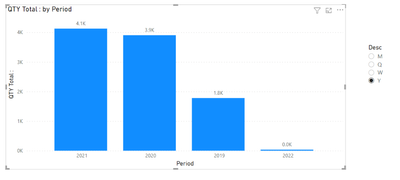2) If I click to “W” or “Q” or “M” no values show up in the chart: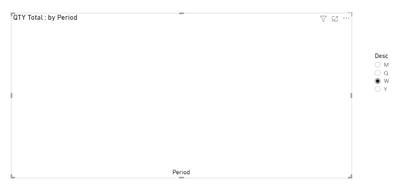3) I have looked at the DAX for the measure “QTY Total” that is used for the chart: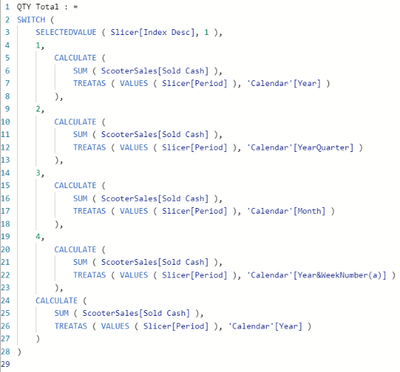4) In my journey to find a solution, I changed the last portion of the DAX by replacing ‘Calendar’[Year] with [Month]:5) With the change, the values for “Y” and "M" appears in the clustered columns visual - but nothing for “Q” or “W"6) How can I modify the DAX to include "Y", "M", "Q" and "W"?

Any help would be appreciated:

https://www.dropbox.com/s/f3hh4vzkkiz0agv/ScooterWorld.pbix?dl=0

1 ACCEPTED SOLUTIONSuper User

Hi @matrix_user
This is one of the simplest and greatest ideas I've ever seen and I really thank you for for posting this.
A small fix and all good to go https://www.dropbox.com/t/vxCj7mCtgVRteRcN

``````QTY Total : =
SWITCH (
SELECTEDVALUE ( Slicer[Index Desc], 1 ),
1,
CALCULATE (
SUM ( ScooterSales[Sold Cash] ),
TREATAS ( VALUES ( Slicer[Period] ), 'Calendar'[Year] )
),
153,
CALCULATE (
SUM ( ScooterSales[Sold Cash] ),
TREATAS ( VALUES ( Slicer[Period] ), 'Calendar'[YearQuarter] )
),
165,
CALCULATE (
SUM ( ScooterSales[Sold Cash] ),
TREATAS ( VALUES ( Slicer[Period] ), 'Calendar'[Month] )
),
5,
CALCULATE (
SUM ( ScooterSales[Sold Cash] ),
TREATAS ( VALUES ( Slicer[Period] ), 'Calendar'[Year&WeekNumber(a)] )
),
CALCULATE (
SUM ( ScooterSales[Sold Cash] ),
TREATAS ( VALUES ( Slicer[Period] ), 'Calendar'[Year] )
)
)``````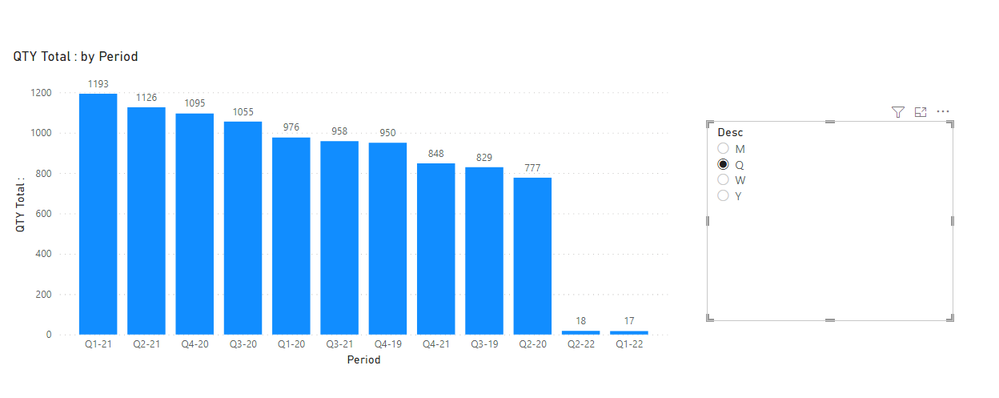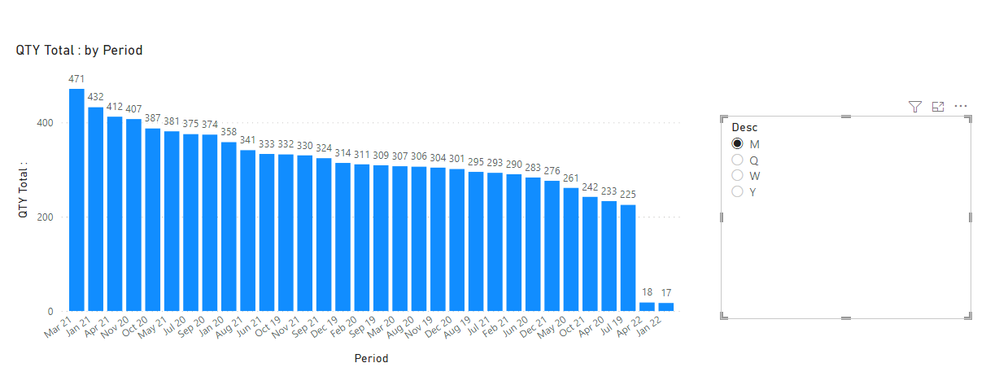4 REPLIES 4Super User

Hi @matrix_user
This is one of the simplest and greatest ideas I've ever seen and I really thank you for for posting this.
A small fix and all good to go https://www.dropbox.com/t/vxCj7mCtgVRteRcN

``````QTY Total : =
SWITCH (
SELECTEDVALUE ( Slicer[Index Desc], 1 ),
1,
CALCULATE (
SUM ( ScooterSales[Sold Cash] ),
TREATAS ( VALUES ( Slicer[Period] ), 'Calendar'[Year] )
),
153,
CALCULATE (
SUM ( ScooterSales[Sold Cash] ),
TREATAS ( VALUES ( Slicer[Period] ), 'Calendar'[YearQuarter] )
),
165,
CALCULATE (
SUM ( ScooterSales[Sold Cash] ),
TREATAS ( VALUES ( Slicer[Period] ), 'Calendar'[Month] )
),
5,
CALCULATE (
SUM ( ScooterSales[Sold Cash] ),
TREATAS ( VALUES ( Slicer[Period] ), 'Calendar'[Year&WeekNumber(a)] )
),
CALCULATE (
SUM ( ScooterSales[Sold Cash] ),
TREATAS ( VALUES ( Slicer[Period] ), 'Calendar'[Year] )
)
)``````Helper III

tamerj1... a big thank you to you!Super User

@matrix_user , I think you trying axis slicer using one of the approaches used in this video

I think you need to use userelationship with few changesHelper III

Hi Amitcanddax,

Nice to know other ways of building dynamic slicers but at this stage DAX is the way to go The DAX shown in the video is leaving me worse that the one I am trying to fix. Cheers